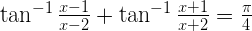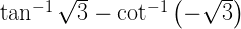NCERT Solutions for Maths Class 12 Exercise 2.2

# NCERT Solutions for Maths Class 12 Exercise 2.2

## NCERT Solutions for Maths Class 12 Exercise 2.2

### Maths Class 12 Ex 2.2 Question 1.

Prove the following.

Solution:
Let sin-1 x = θ
sin θ = x

R.H.S. = sin-1 (3x – 4x³)
= sin-1 (3 sin θ – 4 sin³ θ)
= sin-1 (sin 3θ)                                        [
Since, sin 3θ = 3 sin θ – 4 sin³ θ]
= 3θ
= 3 sin-1 x
= L.H.S.

### Maths Class 12 Ex 2.2 Question 2.

Prove the following.

Solution:

Let cos-1 x = θ
x = cos θ
R.H.S. = cos-1 (4x³ – 3x)
= cos-1 (4 cos³θ – 3 cos θ)
= cos-1 (cos 3θ)                                        [
cos 3θ = 4 cos³ θ – 3 cos θ]
= 3θ
= 3 cos-1 x
= L.H.S.

### Maths Class 12 Ex 2.2 Question 3.

Prove the following.

Solution:

### Maths Class 12 Ex 2.2 Question 10.

Write the following function in the simplest form., a > 0; –a/√3 < x < a/√3

Solution:
Putting x = a tan θ, we get

### Maths Class 12 Ex 2.2 Question 12.

Find the value of the following.

cot [tan-1 a + cot-1 a]

Solution:
Given cot [tan-1 a + cot-1 a]

### Maths Class 12 Ex 2.2 Question 15.

If, then find the value of x.

Solution:

Let sin-1 3/5 = θ, then sin θ = 3/5.

### Maths Class 12 Ex 2.2 Question 19.is equal to
(A) 7π/6
(B) 5π/6
(C) π/3
(D) π/6

Solution:

Hence, option (d) is correct.

### Maths Class 12 Ex 2.2 Question 21.is equal to
(A) π
(B) –π/2

(C) 0
(D) 2√3

Solution: